# Sorting of Arrays in Julia

The process of arranging the given data in a particular order or manner depends on the output. It is storing of data in sorted order. Sorting can be done in two ways i.e in ascending and descending order. Sorting can be performed using sorting algorithm or sorting functions. So, in sorting the program search for minimum number and shift that number to the beginning of the array or list according to the algorithm.

Array plays a very crucial role while sorting. They are used to store the list of the element that has to be sorted.

#### Sorting Techniques in Julia

There are a total of 4(Four) algorithms of sorting in Julia, they are:

• Insertion Sort
• Quick Sort
• PartialQuick Sort(k)
• Merge Sort

#### InsertionSort

This sorting technique traverses the collection one element at a time, inserting each element into its correct, sorted position in the output list.
InsertionSort is an O(n^2) stable sorting algorithm. It is efficient for very small n and is used internally by QuickSort.

#### QuickSort

QuickSort is very fast and mostly used as a default sorting algorithm but it is not stable. Here stable means ” i.e. elements which are considered equal will not remain in the same order in which they originally appeared in the array to be sorted”.
And also as mentioned above it is the default algorithm but only for the numeric value both float and an integer.

On the other hand if we talk about the PartialQuickSort(K) it is similar to QuickSort but the only difference is that the array is sorted up to K if K is an integer or in the range of K.

For example:

 `x ``=` `rand(``1``:``500``, ``100``) `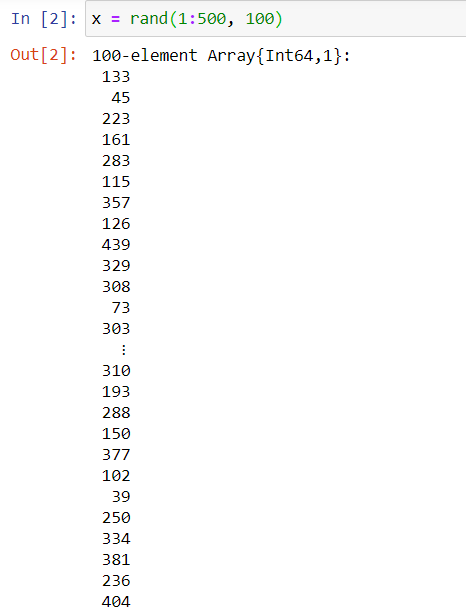so first we have created the random array of 100 elements and stored it in the x.

 `k ``=` `50` `k2 ``=` `50``:``100`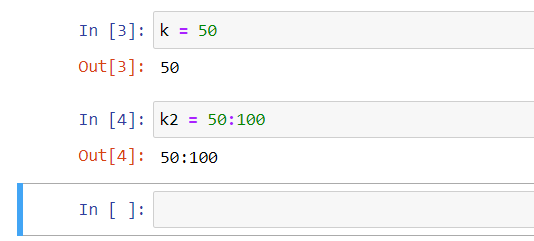Then we have assigned value of k, k2

 `s ``=` `sort(x; alg``=``QuickSort) `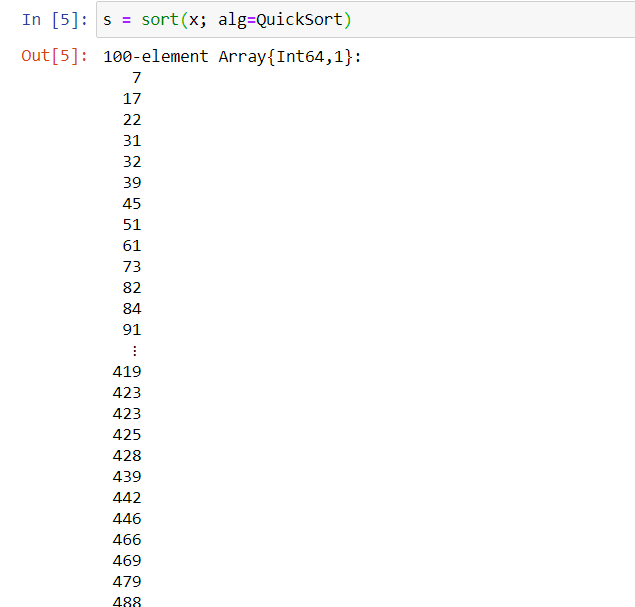Then we have applied QuickSort and sort the array x and you can see the output above.

 `ps ``=` `sort(x; alg``=``PartialQuickSort(k)) ` `qs ``=` `sort(x; alg``=``PartialQuickSort(k2)) `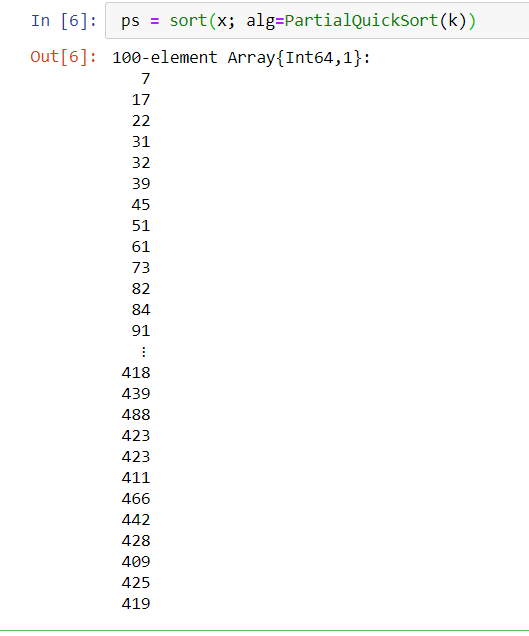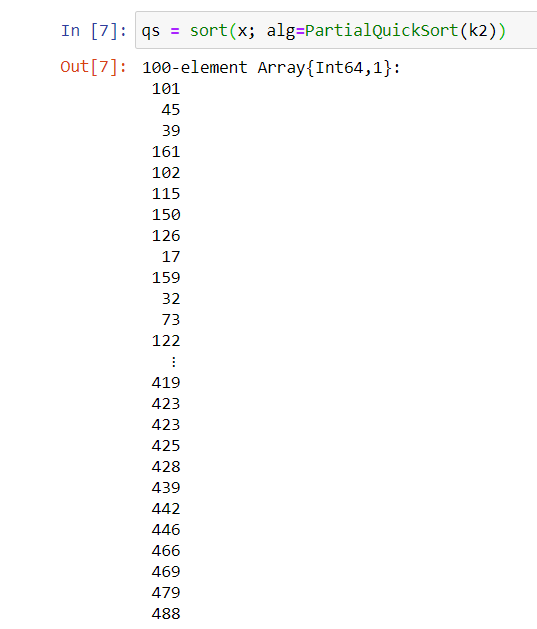Then we used PartialQuickSort with differnt values of k and sorted the array .According to the value of k first we sort the first 50 value of the array and then according to the value of k2 the last 50 values of the array as you can see above

#### MergeSort

Now if we see this sorting algorithm whose complexity is in O(n log n) which is a more stable sorting algorithm. But it needs one more array which is temporary and also which is half the size the given/input array. MergeSort is not as fast as compare to QuickSort. Also it is a default algorithm for non-numeric value.

It totally depends on the programmer which sorting algorithm he wants to use and he can change according to him/her in the syntax.

==>The default algorithm is chosen on the basis that they are fast and stable. As mentioned QuickSort is selected as it is faster. The stability property comes at a non-negligible cost, so if you don’t need it, you may want to explicitly specify your preferred algorithm,

 `sort!(v, alg``=``QuickSort) `

==>The mechanism by which Julia picks default sorting algorithms is implemented via the `Base.Sort.defalg()` function. It allows a particular algorithm to be registered as the default in all sorting functions for specific arrays. For example, here are the two default methods from sort.jl:

 `defalg(v::AbstractArray) ``=` `MergeSort ` `defalg(v::AbstractArray{<:Number}) ``=` `QuickSort `

==>As for numeric arrays, choosing a non-stable default algorithm for array types for which the notion of a stable sort is meaningless (i.e. when two values comparing equal can not be distinguished) may make sense.

### Functions used for Sorting

#### sort() Function

This function returns a sorted array or sorted copy of an array. Let’s understand it more clearly with the help of an example:

Example 1:

 `sort([``2``, ``3``, ``1``]) `

Output: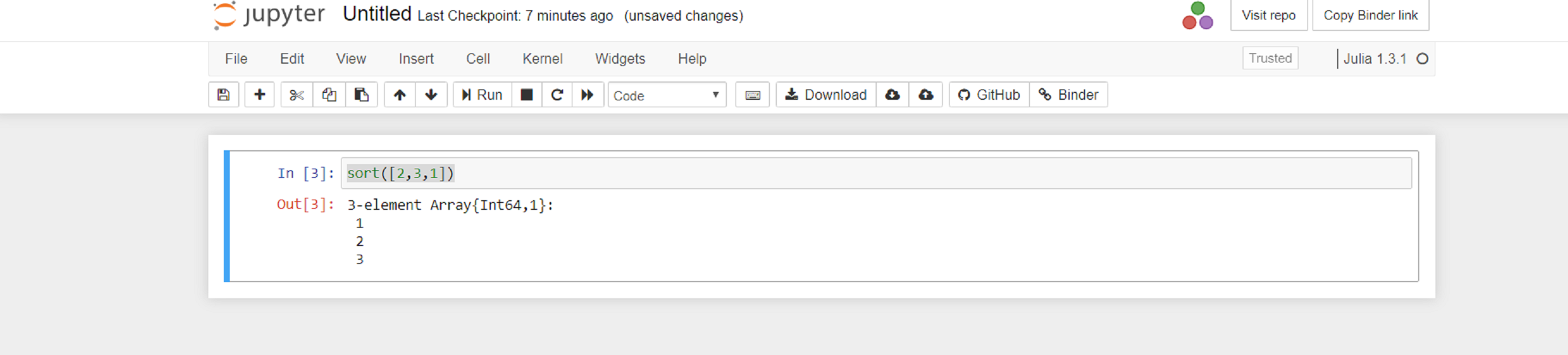In the above example we can clearly see that we have passed the unsorted array in sort function and in return we get the copy of the sorted array.

And also sorting an array in reverse can be done in Julia.

Example 2:

 `sort([``2``, ``3``, ``1``], rev``=``true) `

Output: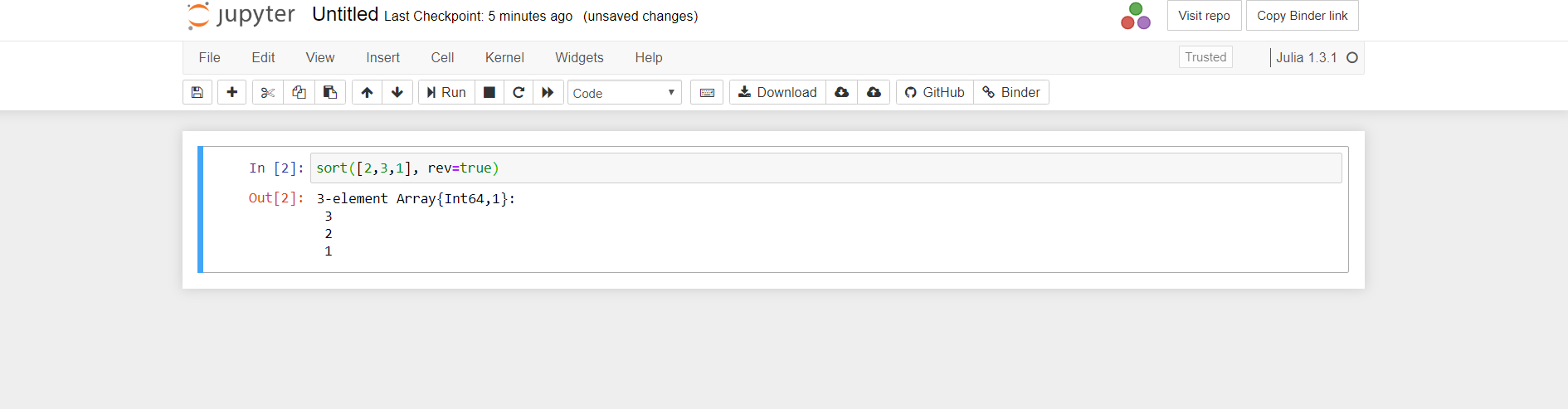#### sortperm() Function

This function also works like `sort()` but instead of returning the copy of it returns a list of indices that one can use to the collection to produce a sorted collection.

Example:

 `r ``=` `rand(``100``:``110``, ``10``) ` `sortperm(r) `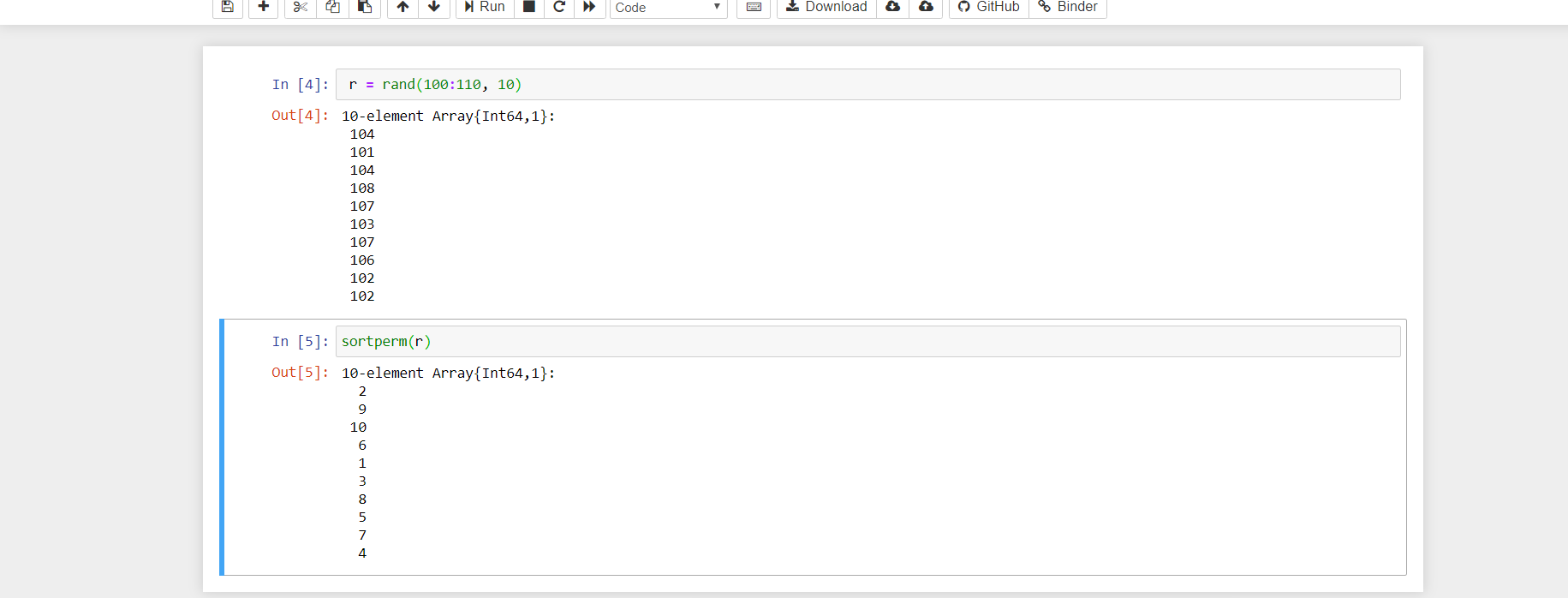Let’s understand the above example properly so in the above example there is a function `rand()` which is used to generate random numbers. We can pass any datatype in the rand function.

Syntax:

`rand([rng=GLOBAL_RNG], [S], [dims...])`

Then when we pass the collection in `sortperm()` then we can observe first output where the indices are generated and these indices are then passed once again as shown in the example we can obtain the desired output.

#### sortrows()

Lexicographical sorting is done on matrix rows using this function. Let’s checkout examples on this to understand:

 `sortrows([``7` `3` `5``; ``-``1` `6` `4``; ``9` `-``2` `8``]) `

Output:

```3×3 Array{Int64, 2}:
-1   6  4
7   3  5
9  -2  8```

 `sortrows([``7` `3` `5``; ``-``1` `6` `4``; ``9` `-``2` `8``], lt``=``(x, y)``-``>isless(x[``2``], y[``2``])) `

Output:

```3×3 Array{Int64, 2}:
9  -2  8
7   3  5
-1   6  4```

 `sortrows([``7` `3` `5``; ``-``1` `6` `4``; ``9` `-``2` `8``], rev``=``true) `

Output:

```3×3 Array{Int64, 2}:
9  -2  8
7   3  5
-1   6  4```

#### sortcols()

Lexicographical sorting is done on matrix rows using this function.

Example:

 `sortcols([``7` `3` `5``; ``6` `-``1` `-``4``; ``9` `-``2` `8``]) `

Output:

```3×3 Array{Int64, 2}:
3   5  7
-1  -4  6
-2   8  9```

 `sortcols([``7` `3` `5``; ``6` `-``1` `-``4``; ``9` `-``2` `8``],  ` `         ``alg``=``InsertionSort, lt``=``(x, y)``-``>isless(x[``2``], y[``2``])) `

Output:

```3×3 Array{Int64, 2}:
5   3  7
-4  -1  6
8  -2  9```

 `sortcols([``7` `3` `5``; ``6` `-``1` `-``4``; ``9` `-``2` `8``], rev``=``true) `

Output:

```3×3 Array{Int64, 2}:
7   5   3
6  -4  -1
9   8  -2```

Now, the big question is what if someone wants to define their own function while sorting the collection other than `sort()` function.

Answer to the above statement is that by using ‘by’ and ‘lt’ keyword.

#### Sort by and Comparison

So, by using the ‘by’ and ‘lt’ keyword one can provide their own function and also compare the elements during sorting.

sort by : This function processes each element before comparison and provides a key for sorting.

Example:

 `r ``=` `[``"1E10"``, ``"150"``, ``"25"``, ``"3"``, ``"1.5"``, ``"1E-10"``, ``"0.5"``, ``".999"``]; `

Using default sort for the above example then it will sort the array in the order in which the character appears in Unicode.

 `sort(r) `

Output: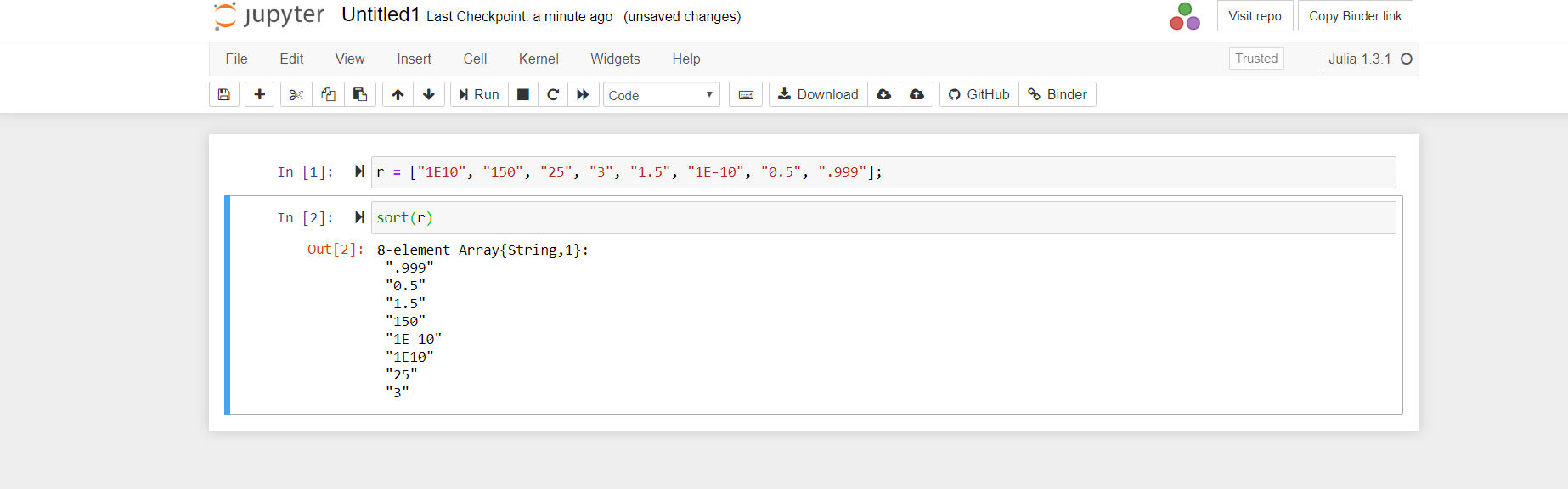“1E-10” appearing after “0.999”.

To sort the numbers by their value, pass the `parse()` function to by:

 `sort(r, by ``=` `x ``-``> Meta.parse(x)) `

Output: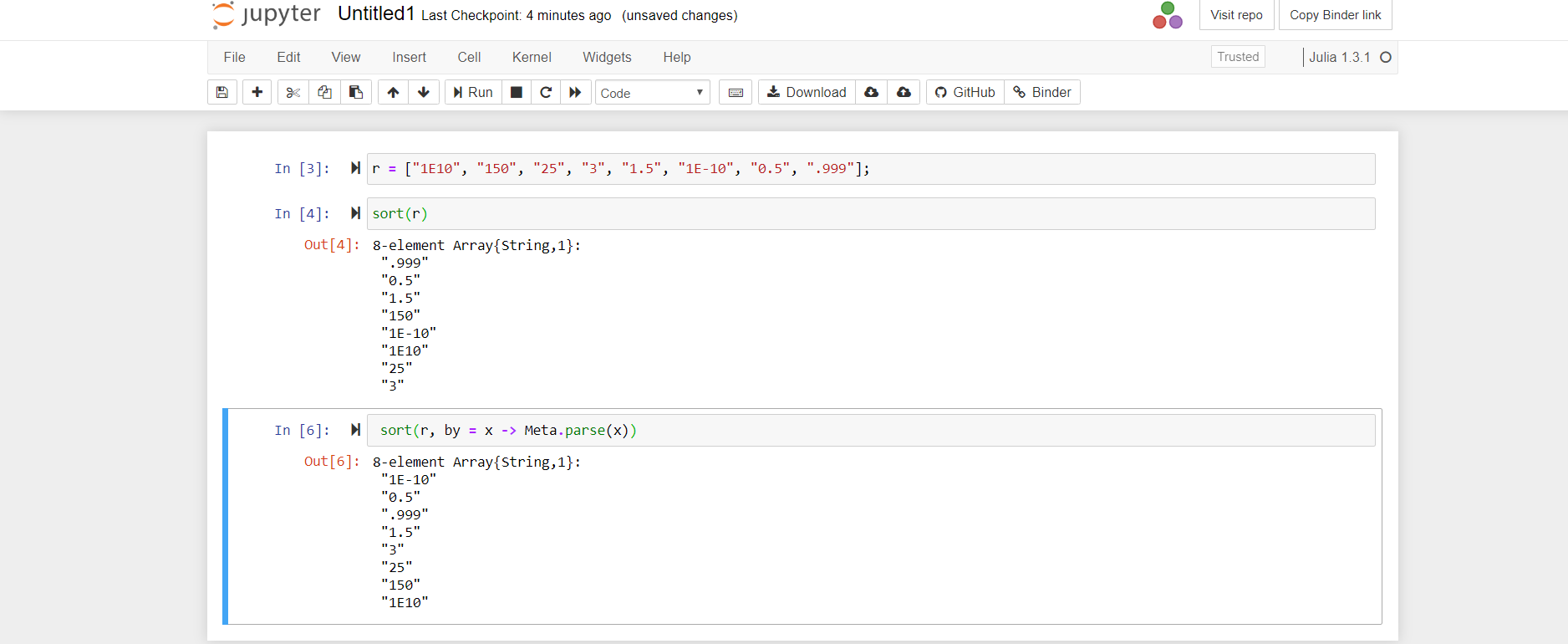The strings are sorted ‘by’ their value.The by function you supply produces the numerical sort key, but the original string elements appear in the final result.

You can also use the anonymous function while sorting.

‘less than’ function:

By default, `isless()` is used while sorting when comparing elements. But, the first element is less than the second in a sorted array.

`'lt'` function is also used to change the behavior by passing the different functions that can be used to compare two elements and return true if they are sorted.

Let’s assume we want to sort an array of words according to the number in each word.

Example , the word “orange” will be considered to be “less than” the word “lemon”, because it has more vowels.

First we’ll need a function that counts vowels:

`vowelcount(string) = count(c -> (c in "aeiou"), lowercase(string))`

Now you can pass an anonymous function to `sort()` that compares the vowel count of two elements using this function and then returns the element with a higher count in each case:

 `sentence ``=` `split("Lorem ipsum dolor sit amet,  ` `consectetur adipisicing elit,  ` `sed do eiusmod tempor incididunt ut labore et dolore magna aliqua."); ` ` `  `sort(sentence, lt ``=` `(x, y) ``-``> vowelcount(x) > vowelcount(y)) `

The result is that the word with the most vowels appears first: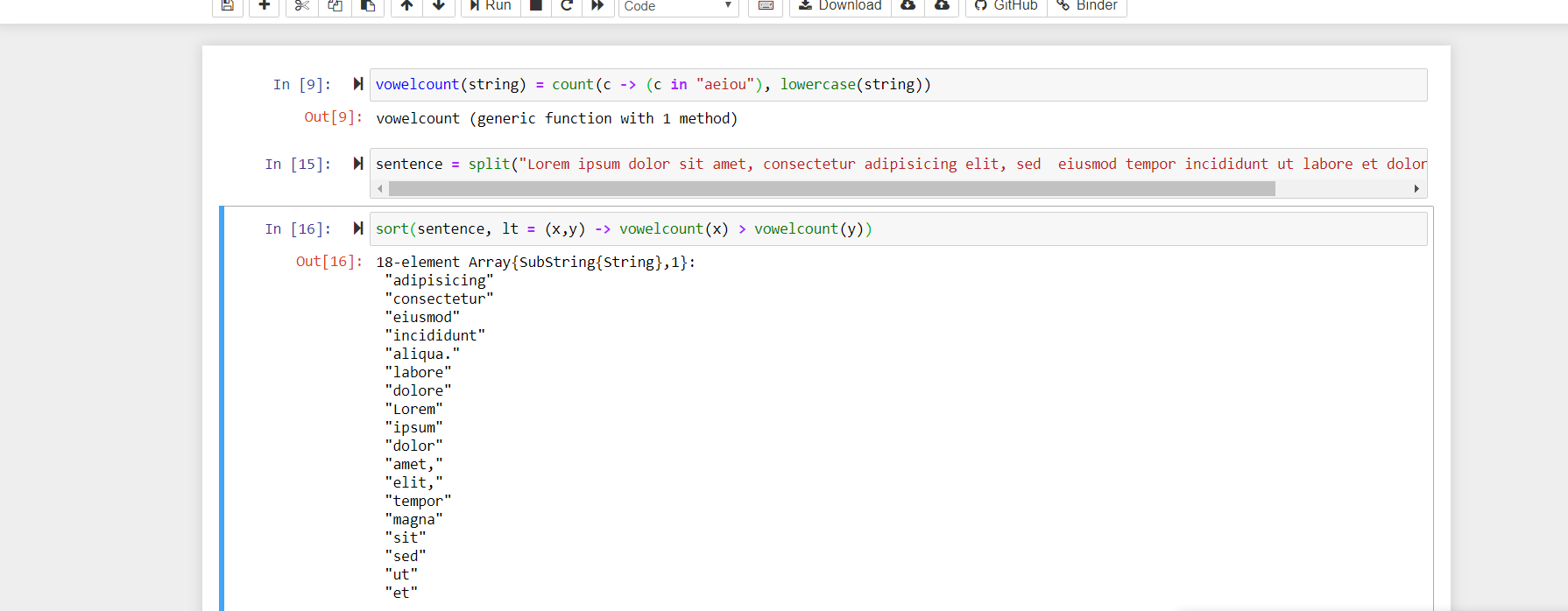The `sort()` function also lets you specify a reverse sort – after the ‘by’ and ‘lt’ functions (if used) have done their work, a true value passed to rev reverses the result.

#### Sorting 2-D Arrays

Using `sortslices()` we can sort a multidimensional array in Julia.

For Example:

 `table ``=` `[``"F"` `"B"` `"I"``; ``"A"` `"D"` `"G"``; ``"H"` `"C"` `"E"``] `

Output:

```3×3 Array{String, 2}:
"F"  "B"  "I"
"A"  "D"  "G"
"H"  "C"  "E"```

You supply a number or a tuple to the dims (“dimensions”) keyword that indicates what you want to sort. To sort the table so that the first column is sorted, use 1:

 `sortslices(table, dims``=``1``) `

Output:

```3×3 Array{String, 2}:
"A"  "D"  "G"
"F"  "B"  "I"
"H"  "C"  "E"```

Note that sortslices returns a new array. The first column is in alphabetical order.

Use dims=2 to sort the table so that the first row is sorted:

 `sortslices(table, dims``=``2``) `

Output:

```3×3 Array{String, 2}:
"B"  "F"  "I"
"D"  "A"  "G"
"C"  "H"  "E"```

Now the first row is in alphabetical order.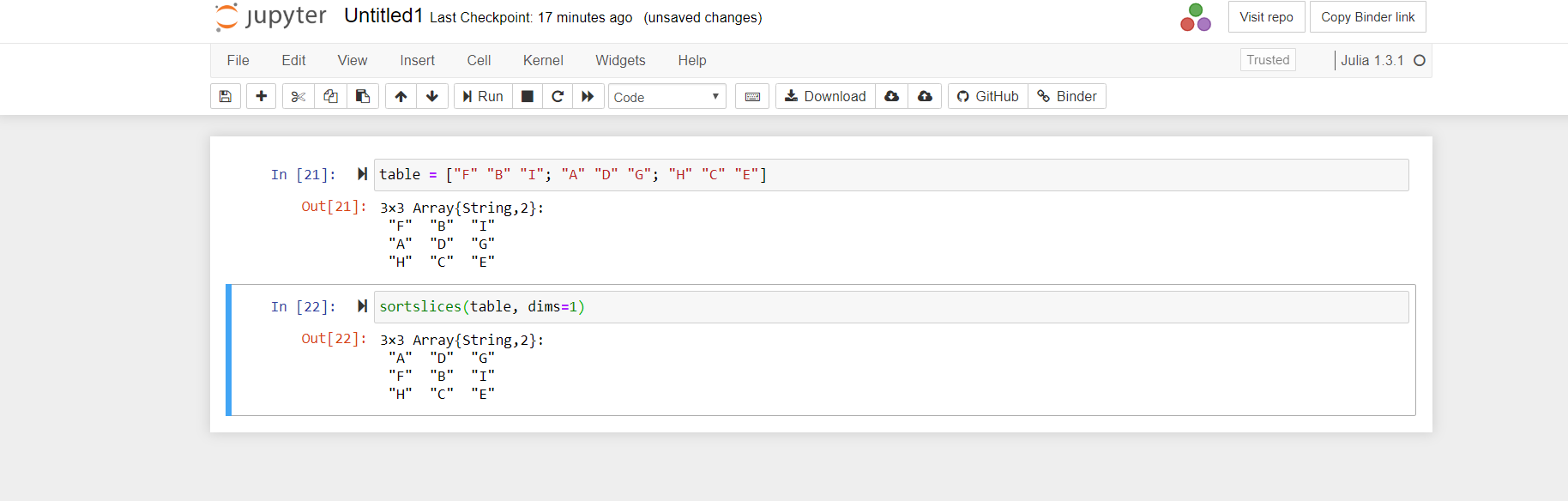If you want to sort by something other than the first item, pass a function to by. So, to sort rows so that the middle column is in alphabetical order, use:

 `sortslices(table, dims``=``1``, by ``=` `x ``-``> x[``2``]) `

Output:

```3×3 Array{String, 2}:
"F"  "B"  "I"
"H"  "C"  "E"
"A"  "D"  "G"```My Personal Notes arrow_drop_upCheck out this Author's contributed articles.

If you like GeeksforGeeks and would like to contribute, you can also write an article using contribute.geeksforgeeks.org or mail your article to contribute@geeksforgeeks.org. See your article appearing on the GeeksforGeeks main page and help other Geeks.

Please Improve this article if you find anything incorrect by clicking on the "Improve Article" button below.

Article Tags :

Be the First to upvote.

Please write to us at contribute@geeksforgeeks.org to report any issue with the above content.